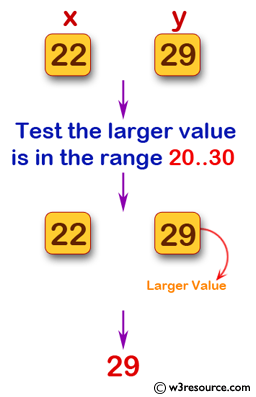﻿ Swift Basic Programming Exercise: Accept two positive integer values and test whether the larger value is in the range 20..30 inclusive - w3resource# Swift Basic Programming Exercise: Accept two positive integer values and test whether the larger value is in the range 20..30 inclusive

## Swift Basic Programming: Exercise-17 with Solution

Write a Swift program that accept two positive integer values and test whether the larger value is in the range 20..30 inclusive, or return 0 if neither is in that range.

Pictorial Presentation:Sample Solution:

Swift Code:

``````func max2030(_ x: Int, _ y: Int) -> Int {
if (x >= 20 && x <= 30 && y >= 20 && y <= 30) && x == y {
return x
} else if x >= 20 && x <= 30 && x > y {
return x
} else if y >= 20 && y <= 30 && y > x {
return y
} else if x >= 20 && x <= 30 && y > 30 {
return x
} else if y >= 20 && y <= 30 && x > 30 {
return y
} else {
return 0
}
}
print(max2030(22, 29))
print(max2030(28, 17))
print(max2030(8, 47))
```
```

Sample Output:

```29
28
0
```

Swift Programming Code Editor:

Improve this sample solution and post your code through Disqus

What is the difficulty level of this exercise?

﻿